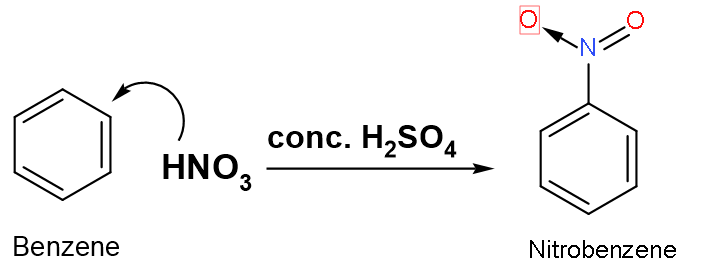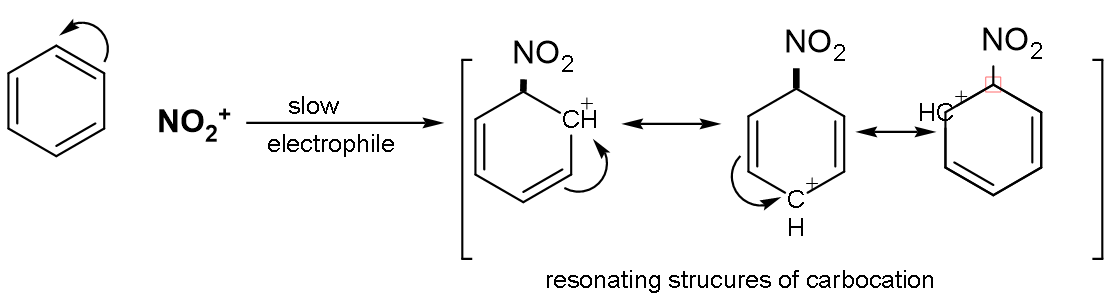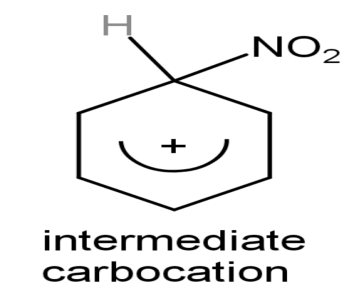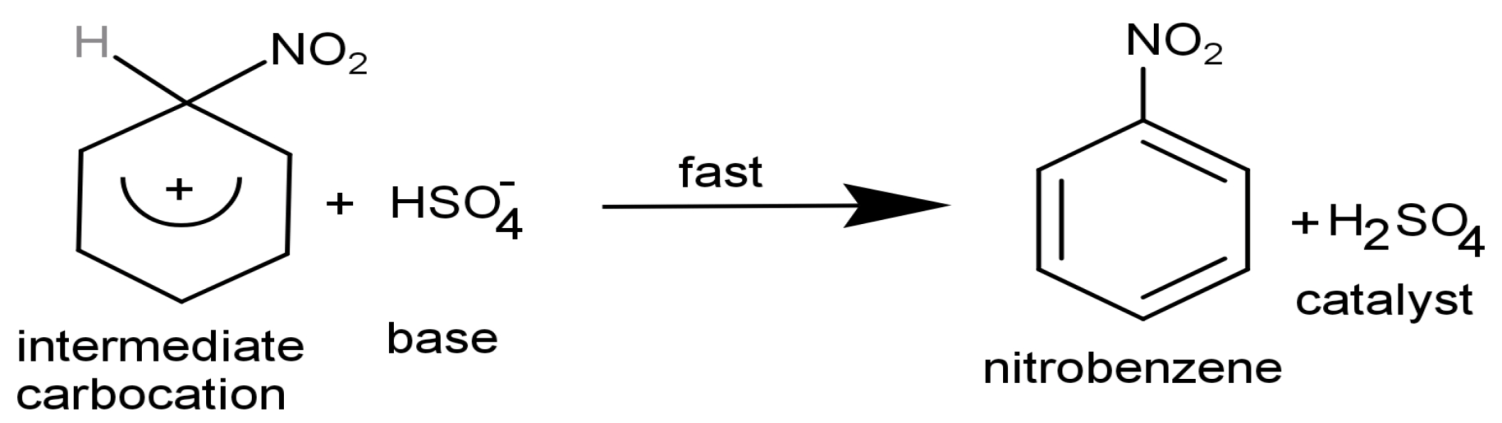QuestionAnswers

# Nitration of benzene is carried out with conc. $\text{HN}{{\text{O}}_{3}}$ in presence of conc.${{\text{H}}_{2}}\text{S}{{\text{O}}_{4}}$. The role of conc. ${{\text{H}}_{2}}\text{S}{{\text{O}}_{4}}$ is to provide:A. nucleophile during the reactionB. free radical during the reactionC. electrophile during the reactionD. catalyst during the reactionVerified
129k+ views
Hint: The role of ${{\text{H}}_{2}}\text{S}{{\text{O}}_{4}}$ in nitration of benzene would be easily understood by looking at the mechanism of nitration on benzene. The product and by-products formed, catalyst involved in the reaction, the type of reaction nitration of benzene is everything could be easily framed with mechanism.

Nitration of benzene is a type of electrophilic substitution reaction. A substitution reaction in which aromatic compounds hydrogen of benzene nucleus is replaced with a nitro group of concentrated nitric acid in presence of concentrated sulphuric acid which yields nitro derivatives is known as ‘nitration’. The ratio of concentrated nitric acid and concentrated sulphuric acid is (1:2) in the mixture as reagents of the reaction.Let us look at the mechanism of the reaction:
Step (1): Formation of electrophile: In nitration, nitronium ion is the electrophile. It is produced by the reaction of nitric acid with sulphuric acid.
$\text{HN}{{\text{O}}_{3}}+\text{2}{{\text{H}}_{2}}\text{S}{{\text{O}}_{4}}\to \overset{+}{\mathop{\text{N}}}\,{{\text{O}}_{2}}+{{\text{H}}_{3}}{{\text{O}}^{+}}+\text{2HS}{{\text{O}}_{4}}^{-}$, where $\overset{+}{\mathop{\text{N}{{\text{O}}_{2}}}}\,$is the electrophile.
Step (2): Formation of carbonium ion: On attacking of electrophile which is$\overset{+}{\mathop{\text{N}{{\text{O}}_{2}}}}\,$on the benzene nucleus, the intermediate carbocation is formed.The intermediate carbonium ion isStep (3): Formation of final product: On attacking the nucleophile that is on the intermediate carbocation and giving the final product by attraction of the proton.Thus, the concentrated sulphuric acid or ${{\text{H}}_{2}}\text{S}{{\text{O}}_{4}}$ is acting as a catalyst in nitration reaction with benzene as it is obtained back by the end of the reaction.
So, the correct answer is “Option D”.
Note:
The resonating structures should be made correctly and carefully. The main step is to obtain the electrophile which will be substituted on the benzene. Electrophile does not mean the one with a positive sign on it but the one whose orbitals are empty or electron deficient, like $\text{S}{{\text{O}}_{3}}$. It is an electrophile without having positive charge on its head.